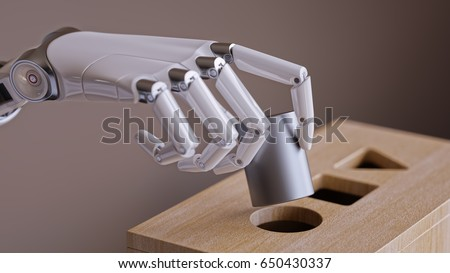# Neural Net Vs MNIST (Part 2)

In part 1 we trained a 1 layer neural net to predict the correct digit when shown a 28×28 gray scale image of a handwritten digit. After several thousand training steps the network eventually managed to get an average test error of ~10%. In this post I would like to look under the hood of the network and investigate what it actually learned, how it decides between different classes. We will also see why despite having 90% accuracy on the test set the network is not very useful for solving any actual real world classification tasks.

## Can the model classify my handwritten digits ?

Running the model over the test set is one thing, but I wanted something more interactive. I therefore created a small python application that allows me to draw on screen. The application takes the drawing and presents it to the imported tensorflow model from part 1 in the same format as all the other MNIST images the model was trained on. Based on this information the model will try and predict the type of digit I drew and tell me how certain it is about its prediction.

The first impression is that the model does a good job of recognizing the digits, it does struggle with the digits 7 and 9, but that is understandable as a 7 looks similar to a 1 and a 9 shares a lot of characteristics with a 8 etc. What I have not shown in the video above however is that the model breaks down completely if the digits differ only slightly from the way the digit is drawn on average in the MNIST training set. Below you can see how the model fails to identify the correct class despite the digits in the input being very similar.The same effect can be seen when the style of the pen used for drawing is changed (e.g. thicker or thinner). Any deviations (even just slight ones) from the digits in the MNIST dataset and the model fails. The model cannot generalize, meaning that it cannot apply learned concepts to new input data. This is because the model has not actually learned what a 5 is (a horizontal line at the top, then a vertical line, and then a kind of half circle), all the model is essentially doing is taking a set of planks with cut out shapes and seeing through which hole the input shape fits best (similar to those shape sorting toys you can buy for children).This works well if the input shapes have the right size, but if a cylinder is suddenly twice as big as the circular hole (or slightly oval), but fits through the square hole, the model will think that it must be a cube since it fits so nicely through the square hole. So, can the model classify my handwritten digits ? Sort of … but not really.

## Looking at what the model has learned.

To show the problem I described in the previous section I modified the plotting section of the module that is responsible for the training of the model so that it shows the weight vector for each neuron instead. Since each neuron has a weight connected to each pixel of the input its easily possible to show the weight vector as an image.The top row of the image shows the weights associated with each neuron (title above each plot indicates what digit this neuron is responsible for detecting). Brighter pixels mean that the corresponding position in the input image has a larger importance when it comes to determining if the input is the digit that the neuron is looking for (and vice versa). This illustrates well the idea with the shapes described above. By looking at a plot it is now easy to see why the smaller 5 at the top left corner in the previous section got wrongly classified, it simply overlapped with more of the bright pixels from the 7 neuron than it did with pixels from the 5 neuron.

The rows with “delta_” in the title show the weight change since the last plot update. You can see several numbers overlapping here since a batch size of 2 means that two inputs are applied before the weights are changed. In the video below you can see how the weights change as the model trains. the delta plots become less clear towards the end because I am increasing the steps the model does before the graph is refreshed, so multiple update cycles get included in one plot (did this to speed up the training in the demo).

I will soon make another post on classifying handwritten digits but using a convolutional neural net to show the advantages of that architecture. The code for everything shown in this post can be found on my github. If you did/didn’t enjoy this and/or have suggestions please leave some feedback below 🙂 .